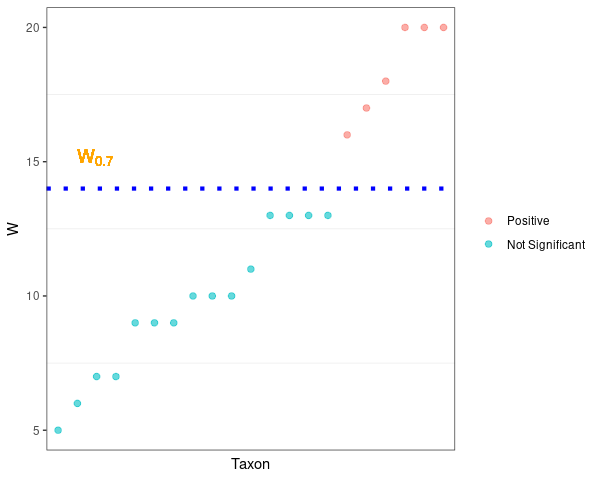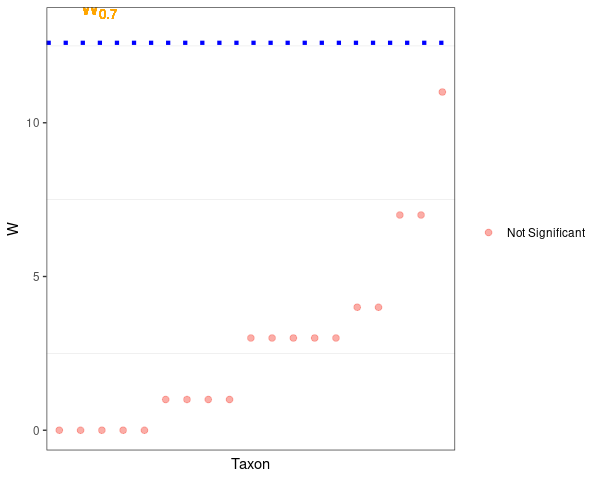# ANCOM Tutorial

knitr::opts_chunk$set(message = FALSE, warning = FALSE, comment = NA, fig.width = 6.25, fig.height = 5) library(ANCOMBC) library(tidyverse) # 1. Introduction Analysis of Composition of Microbiomes (ANCOM) (Mandal et al. 2015) is a differential abundance (DA) analysis for microbial absolute abundances. It accounts for the compositionality of microbiome data by performing the additive log ratio (ALR) transformation. ANCOM employs a heuristic strategy to declare taxa that are significantly differentially abundant. For a given taxon, the output W statistic represents the number ALR transformed models where the taxon is differentially abundant with regard to the variable of interest. The larger the value of W, the more likely the taxon is differentially abundant. For more details, please refer to the ANCOM paper. # 2. Installation Download package. if (!requireNamespace("BiocManager", quietly = TRUE)) install.packages("BiocManager") BiocManager::install("ANCOMBC") Load the package. library(ANCOMBC) # 3. Run ANCOM on a real cross-sectional dataset ## 3.1 Import example data The HITChip Atlas dataset contains genus-level microbiota profiling with HITChip for 1006 western adults with no reported health complications, reported in (Lahti et al. 2014). The dataset is available via the microbiome R package (Lahti et al. 2017) in phyloseq (McMurdie and Holmes 2013) format. In this tutorial, we consider the following covariates: • Continuous covariates: “age” • Categorical covariates: “region”, “bmi” • The group variable of interest: “bmi” • Three groups: “lean”, “overweight”, “obese” • The reference group: “obese” data(atlas1006, package = "microbiome") tse = mia::makeTreeSummarizedExperimentFromPhyloseq(atlas1006) # Subset to baseline tse = tse[, tse$time == 0]

# Re-code the bmi group
tse$bmi = recode(tse$bmi_group,
obese = "obese",
severeobese = "obese",
morbidobese = "obese")
# Subset to lean, overweight, and obese subjects
tse = tse[, tse$bmi %in% c("lean", "overweight", "obese")] # Note that by default, levels of a categorical variable in R are sorted # alphabetically. In this case, the reference level for bmi will be # lean. To manually change the reference level, for instance, setting obese # as the reference level, use: tse$bmi = factor(tse$bmi, levels = c("obese", "overweight", "lean")) # You can verify the change by checking: # levels(sample_data(tse)$bmi)

# Create the region variable
tse$region = recode(as.character(tse$nationality),
Scandinavia = "NE", UKIE = "NE", SouthEurope = "SE",
CentralEurope = "CE", EasternEurope = "EE",
.missing = "unknown")

# Discard "EE" as it contains only 1 subject
# Discard subjects with missing values of region
tse = tse[, ! tse$region %in% c("EE", "unknown")] print(tse) class: TreeSummarizedExperiment dim: 130 873 metadata(0): assays(1): counts rownames(130): Actinomycetaceae Aerococcus ... Xanthomonadaceae Yersinia et rel. rowData names(3): Phylum Family Genus colnames(873): Sample-1 Sample-2 ... Sample-1005 Sample-1006 colData names(12): age sex ... bmi region reducedDimNames(0): mainExpName: NULL altExpNames(0): rowLinks: NULL rowTree: NULL colLinks: NULL colTree: NULL ## 3.2 Run ancom function set.seed(123) out = ancom(data = tse, assay_name = "counts", tax_level = "Family", phyloseq = NULL, p_adj_method = "holm", prv_cut = 0.10, lib_cut = 1000, main_var = "bmi", adj_formula = "age + region", rand_formula = NULL, lme_control = NULL, struc_zero = TRUE, neg_lb = TRUE, alpha = 0.05, n_cl = 2) res = out$res

# Similarly, if the main variable of interest is continuous, such as age, the
# ancom model can be specified as
# out = ancom(data = tse, assay_name = "counts",
#             tax_level = "Family", phyloseq = NULL,
#             p_adj_method = "holm", prv_cut = 0.10, lib_cut = 1000,
#             main_var = "age", adj_formula = "bmi + region",
#             rand_formula = NULL, lme_control = NULL, struc_zero = FALSE,
#             neg_lb = FALSE, alpha = 0.05, n_cl = 2)

# ancom also supports importing data in phyloseq format
# tse_alt = agglomerateByRank(tse, "Family")
# pseq = makePhyloseqFromTreeSummarizedExperiment(tse_alt)
# out = ancom(data = NULL, assay_name = NULL,
#             tax_level = "Family", phyloseq = pseq,
#             p_adj_method = "holm", prv_cut = 0.10, lib_cut = 1000,
#             main_var = "bmi", adj_formula = "age + region",
#             rand_formula = NULL, lme_control = NULL, struc_zero = TRUE,
#             neg_lb = TRUE, alpha = 0.05, n_cl = 2)

## 3.3 Scatter plot for W statistics

q_val = out$q_data beta_val = out$beta_data
# Only consider the effect sizes with the corresponding q-value less than alpha
beta_val = beta_val * (q_val < 0.05)
# Choose the maximum of beta's as the effect size
beta_pos = apply(abs(beta_val), 2, which.max)
beta_max = vapply(seq_along(beta_pos), function(i)
beta_val[beta_pos[i], i], FUN.VALUE = double(1))
# Number of taxa except structural zeros
n_taxa = ifelse(is.null(out$zero_ind), nrow(tse), sum(apply(out$zero_ind[, -1], 1, sum) == 0))
# Cutoff values for declaring differentially abundant taxa
cut_off = 0.7 * (n_taxa - 1)

df_fig_w = res %>%
dplyr::mutate(beta = beta_max,
direct = case_when(
detected_0.7 == TRUE & beta > 0 ~ "Positive",
detected_0.7 == TRUE & beta <= 0 ~ "Negative",
TRUE ~ "Not Significant"
)) %>%
dplyr::arrange(W)
df_fig_w$taxon = factor(df_fig_w$taxon, levels = df_fig_w$taxon) df_fig_w$W = replace(df_fig_w$W, is.infinite(df_fig_w$W), n_taxa - 1)
df_fig_w$direct = factor(df_fig_w$direct,
levels = c("Negative", "Positive", "Not Significant"))

p_w = df_fig_w %>%
ggplot(aes(x = taxon, y = W, color = direct)) +
geom_point(size = 2, alpha = 0.6) +
labs(x = "Taxon", y = "W") +
scale_color_discrete(name = NULL) +
geom_hline(yintercept = cut_off, linetype = "dotted",
color = "blue", size = 1.5) +
geom_text(aes(x = 2, y = cut_off + 0.5, label = "W[0.7]"),
size = 5, vjust = -0.5, hjust = 0, color = "orange", parse = TRUE) +
theme_bw() +
theme(axis.text.x = element_blank(),
axis.ticks.x = element_blank(),
panel.grid.major = element_blank())
p_w# 4. Run ANCOM on a real longitudinal dataset

## 4.1 Import example data

A two-week diet swap study between western (USA) and traditional (rural Africa) diets (Lahti et al. 2014). The dataset is available via the microbiome R package (Lahti et al. 2017) in phyloseq (McMurdie and Holmes 2013) format. In this tutorial, we consider the following fixed effects:

• Continuous covariates: “timepoint”

• Categorical covariates: “nationality”

• The group variable of interest: “group”

• Three groups: “DI”, “ED”, “HE”

• The reference group: “DI”

and the following random effects:

• A random intercept

• A random slope: “timepoint”

data(dietswap, package = "microbiome")
tse = mia::makeTreeSummarizedExperimentFromPhyloseq(dietswap)
print(tse)
class: TreeSummarizedExperiment
dim: 130 222
assays(1): counts
Yersinia et rel.
rowData names(3): Phylum Family Genus
colnames(222): Sample-1 Sample-2 ... Sample-221 Sample-222
colData names(8): subject sex ... timepoint.within.group bmi_group
reducedDimNames(0):
mainExpName: NULL
altExpNames(0):
rowTree: NULL
colTree: NULL

## 4.2 Run ancom function

set.seed(123)
out = ancom(data = tse, assay_name = "counts",
tax_level = "Family", phyloseq = NULL,
p_adj_method = "holm", prv_cut = 0.10, lib_cut = 1000,
main_var = "group",
rand_formula = "(timepoint | subject)",
lme_control = lme4::lmerControl(),
struc_zero = TRUE, neg_lb = TRUE, alpha = 0.05, n_cl = 2)

res = out$res ## 4.3 Visualization for W statistics q_val = out$q_data
beta_val = out$beta_data # Only consider the effect sizes with the corresponding q-value less than alpha beta_val = beta_val * (q_val < 0.05) # Choose the maximum of beta's as the effect size beta_pos = apply(abs(beta_val), 2, which.max) beta_max = vapply(seq_along(beta_pos), function(i) beta_val[beta_pos[i], i], FUN.VALUE = double(1)) # Number of taxa except structural zeros n_taxa = ifelse(is.null(out$zero_ind),
nrow(tse),
sum(apply(out$zero_ind[, -1], 1, sum) == 0)) # Cutoff values for declaring differentially abundant taxa cut_off = 0.7 * (n_taxa - 1) df_fig_w = res %>% dplyr::mutate(beta = beta_max, direct = case_when( detected_0.7 == TRUE & beta > 0 ~ "Positive", detected_0.7 == TRUE & beta <= 0 ~ "Negative", TRUE ~ "Not Significant" )) %>% dplyr::arrange(W) df_fig_w$taxon = factor(df_fig_w$taxon, levels = df_fig_w$taxon)
df_fig_w$W = replace(df_fig_w$W, is.infinite(df_fig_w$W), n_taxa - 1) df_fig_w$direct = factor(df_fig_w\$direct,
levels = c("Negative", "Positive", "Not Significant"))

p_w = df_fig_w %>%
ggplot(aes(x = taxon, y = W, color = direct)) +
geom_point(size = 2, alpha = 0.6) +
labs(x = "Taxon", y = "W") +
scale_color_discrete(name = NULL) +
geom_hline(yintercept = cut_off, linetype = "dotted",
color = "blue", size = 1.5) +
geom_text(aes(x = 2, y = cut_off + 0.5, label = "W[0.7]"),
size = 5, vjust = -0.5, hjust = 0, color = "orange", parse = TRUE) +
theme_bw() +
theme(axis.text.x = element_blank(),
axis.ticks.x = element_blank(),
panel.grid.major = element_blank())
p_w# Session information

sessionInfo()
R version 4.3.0 RC (2023-04-13 r84269)
Platform: x86_64-pc-linux-gnu (64-bit)
Running under: Ubuntu 22.04.2 LTS

Matrix products: default
BLAS:   /home/biocbuild/bbs-3.17-bioc/R/lib/libRblas.so
LAPACK: /usr/lib/x86_64-linux-gnu/lapack/liblapack.so.3.10.0

locale:
 LC_CTYPE=en_US.UTF-8       LC_NUMERIC=C
 LC_TIME=en_GB              LC_COLLATE=C
 LC_MONETARY=en_US.UTF-8    LC_MESSAGES=en_US.UTF-8
 LC_PAPER=en_US.UTF-8       LC_NAME=C
 LC_MEASUREMENT=en_US.UTF-8 LC_IDENTIFICATION=C

time zone: America/New_York
tzcode source: system (glibc)

attached base packages:
 stats     graphics  grDevices utils     datasets  methods   base

other attached packages:
 phyloseq_1.44.0 lubridate_1.9.2 forcats_1.0.0   stringr_1.5.0
 tibble_3.2.1    ggplot2_3.4.2   tidyverse_2.0.0 ANCOMBC_2.2.0

loaded via a namespace (and not attached):
 splines_4.3.0                  bitops_1.0-7
 cellranger_1.1.0               rpart_4.1.19
 DirichletMultinomial_1.42.0    lifecycle_1.0.3
 Rdpack_2.4                     doParallel_1.0.17
 lattice_0.21-8                 MASS_7.3-59
 MultiAssayExperiment_1.26.0    backports_1.4.1
 magrittr_2.0.3                 Hmisc_5.0-1
 sass_0.4.5                     rmarkdown_2.21
 jquerylib_0.1.4                yaml_2.3.7
 doRNG_1.8.6                    gld_2.6.6
 DBI_1.1.3                      minqa_1.2.5
 zlibbioc_1.46.0                expm_0.999-7
 GenomicRanges_1.52.0           BiocGenerics_0.46.0
 RCurl_1.98-1.12                yulab.utils_0.0.6
 nnet_7.3-18                    TH.data_1.1-2
 sandwich_3.0-2                 GenomeInfoDbData_1.2.10
 IRanges_2.34.0                 S4Vectors_0.38.0
 ggrepel_0.9.3                  irlba_2.3.5.1
 tidytree_0.4.2                 vegan_2.6-4
 permute_0.9-7                  DelayedMatrixStats_1.22.0
 codetools_0.2-19               DelayedArray_0.26.0
 scuttle_1.10.0                 energy_1.7-11
 tidyselect_1.2.0               farver_2.1.1
 lme4_1.1-33                    gmp_0.7-1
 ScaledMatrix_1.8.0             viridis_0.6.2
 matrixStats_0.63.0             stats4_4.3.0
 base64enc_0.1-3                jsonlite_1.8.4
 multtest_2.56.0                BiocNeighbors_1.18.0
 e1071_1.7-13                   decontam_1.20.0
 mia_1.8.0                      Formula_1.2-5
 survival_3.5-5                 scater_1.28.0
 iterators_1.0.14               emmeans_1.8.5
 foreach_1.5.2                  tools_4.3.0
 treeio_1.24.0                  DescTools_0.99.48
 Rcpp_1.0.10                    glue_1.6.2
 gridExtra_2.3                  xfun_0.39
 mgcv_1.8-42                    MatrixGenerics_1.12.0
 GenomeInfoDb_1.36.0            TreeSummarizedExperiment_2.8.0
 withr_2.5.0                    numDeriv_2016.8-1.1
 fastmap_1.1.1                  rhdf5filters_1.12.0
 boot_1.3-28.1                  fansi_1.0.4
 digest_0.6.31                  rsvd_1.0.5
 timechange_0.2.0               R6_2.5.1
 estimability_1.4.1             colorspace_2.1-0
 RSQLite_2.3.1                  utf8_1.2.3
 generics_0.1.3                 data.table_1.14.8
 DECIPHER_2.28.0                class_7.3-21
 CVXR_1.0-11                    httr_1.4.5
 htmlwidgets_1.6.2              pkgconfig_2.0.3
 gtable_0.3.3                   Exact_3.2
 Rmpfr_0.9-2                    blob_1.2.4
 SingleCellExperiment_1.22.0    XVector_0.40.0
 htmltools_0.5.5                biomformat_1.28.0
 scales_1.2.1                   Biobase_2.60.0
 lmom_2.9                       knitr_1.42
 rstudioapi_0.14                tzdb_0.3.0
 reshape2_1.4.4                 coda_0.19-4
 checkmate_2.1.0                nlme_3.1-162
 nloptr_2.0.3                   rhdf5_2.44.0
 proxy_0.4-27                   cachem_1.0.7
 zoo_1.8-12                     rootSolve_1.8.2.3
 parallel_4.3.0                 vipor_0.4.5
 foreign_0.8-84                 pillar_1.9.0
 grid_4.3.0                     vctrs_0.6.2
 BiocSingular_1.16.0            beachmat_2.16.0
 xtable_1.8-4                   cluster_2.1.4
 beeswarm_0.4.0                 htmlTable_2.4.1
 evaluate_0.20                  mvtnorm_1.1-3
 cli_3.6.1                      compiler_4.3.0
 rlang_1.1.0                    crayon_1.5.2
 rngtools_1.5.2                 labeling_0.4.2
 plyr_1.8.8                     ggbeeswarm_0.7.1
 stringi_1.7.12                 viridisLite_0.4.1
 BiocParallel_1.34.0            lmerTest_3.1-3
 munsell_0.5.0                  Biostrings_2.68.0
 gsl_2.1-8                      lazyeval_0.2.2
 Matrix_1.5-4                   hms_1.1.3
 sparseMatrixStats_1.12.0       bit64_4.0.5
 Rhdf5lib_1.22.0                highr_0.10
 SummarizedExperiment_1.30.0    rbibutils_2.2.13
 igraph_1.4.2                   memoise_2.0.1
 bslib_0.4.2                    bit_4.0.5
 readxl_1.4.2                   ape_5.7-1